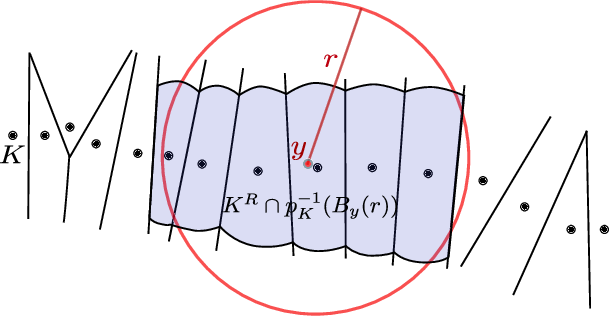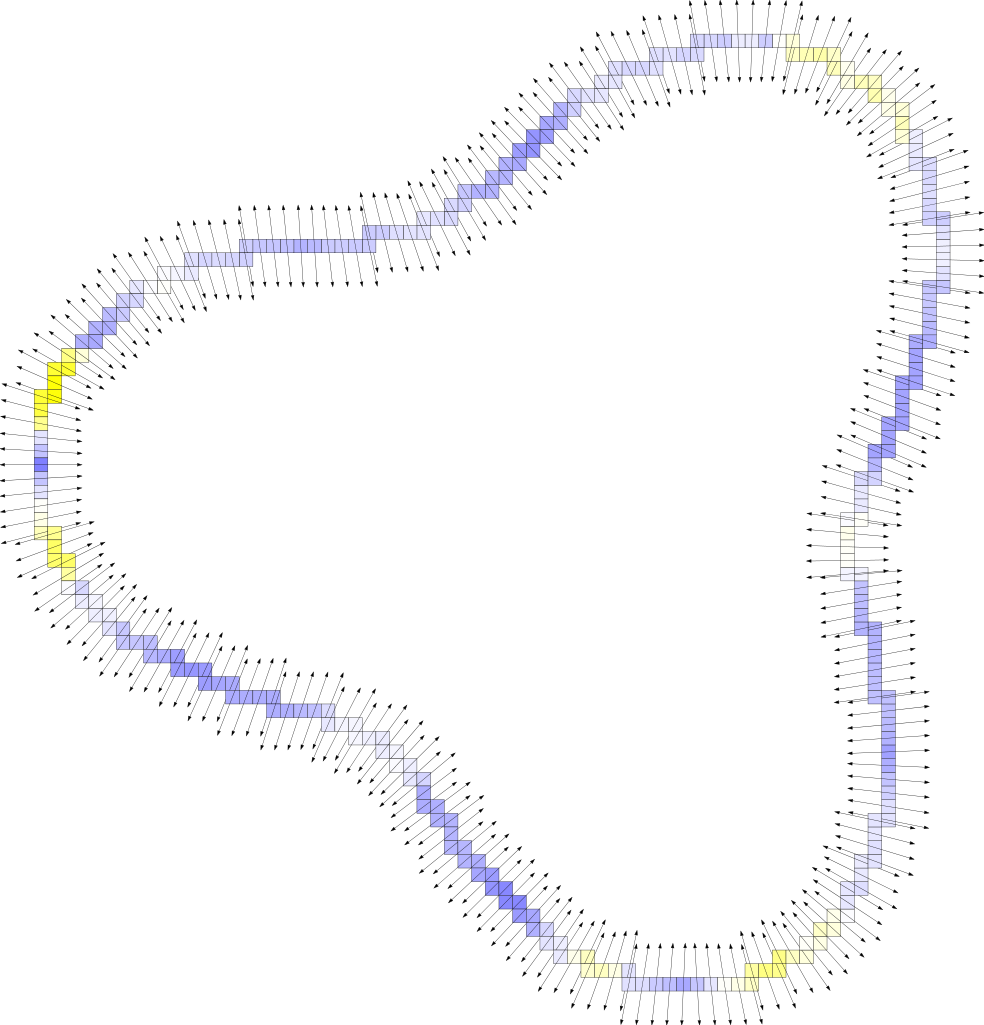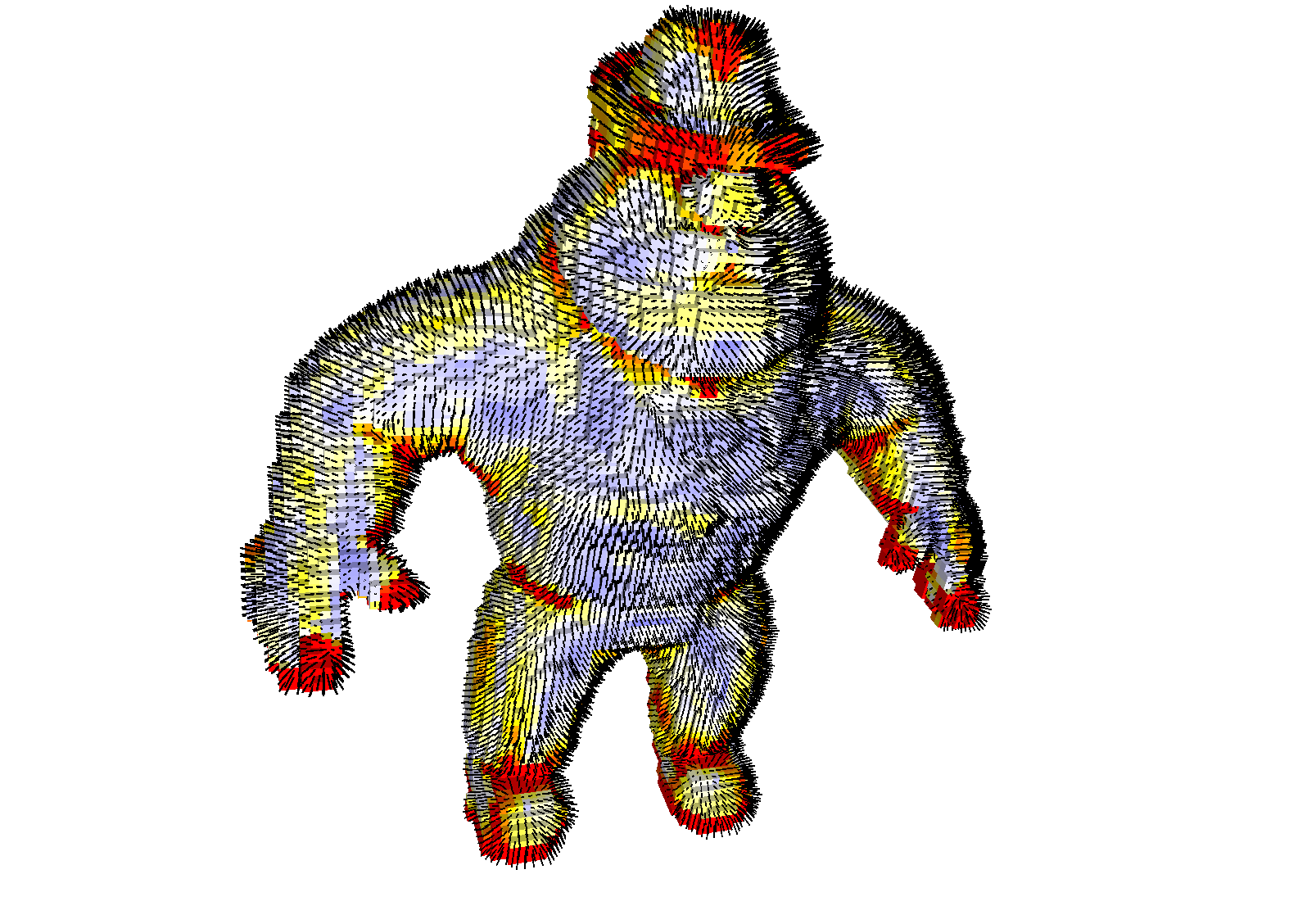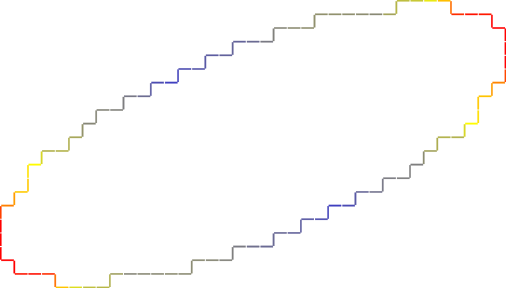DGtal  1.4.beta
Digital Voronoi Covariance Measure and geometry estimation
Author(s) of this documentation:
Jacques-Olivier Lachaud

Part of the Geometry package.

This part of the manual describes classes and functions related to the Voronoi Covariance Measure (VCM) of a set of points in arbitrary dimension. The VCM is a covariance tensor related to a distance function to the input data points. It was introduced by Mérigot, Ovsjanikov and Guibas . The digital version of the VCM was studied by Cuel, Lachaud, and Thibert . Stability and multigrid convergence results have been established. This documentation presents the implementation of the digital VCM. The VCM is useful for the following tasks:

• normal cone estimation: Given a set of digital points approximating a surface, the VCM estimates robustly the normal vector to this underlying surface.
• geometry estimation: Given a digital surface approximating some surface, the VCM can estimate robustly the oriented normal vector and the principal directions of the underlying surface.
• feature detection: Given a set of digital points approximating a surface, the eigenvalues of the VCM can be combined to define a robust feature detector.

# Voronoi Covariance Measure

The Voronoi covariance measure (VCM) has been introduced in  for normals and curvature estimations. Let K be a compact subset of $$\mathbb{R}^3$$ and $$d_K$$ the distance function to K, i.e. the map $$d_K(x) := \min_{p\in K} \|p-x\|$$. A point p where the previous minimum is reached is called a projection of x on K. Almost every point admits a single projection on K, thus definining a map $$p_K: \mathbb{R}^3 \to K$$ almost everywhere. The R-offset of K is the R-sublevel set of $$d_K$$, i.e. the set $$K^R:=d_K^{-1}(]-\infty, R[)$$. The VCM maps any integrable function $$\chi:\mathbb{R}^3 \to \mathbb{R}^+$$ to the matrix

$\mathcal{V}_{K,R}(\chi) := \int_{K^R}(x-p_K(x))(x-p_K(x))^{\mathbf{t}} \chi(p_K(x)) d x.$Voronoi Covariance Measure of a point set in the plane.
Note
The stability result of  implies that information extracted from the covariance matrix such as normals or principal directions are stable with respect to Hausdorff perturbation.
Remark that this definition matches the definition introduced in  : when $$\chi$$ is the characteristic function of a ball, one recovers a notion similar to the convolved VCM.

# Computing the Voronoi Covariance Measure of a point set

Let K be some point set. In this case, the computation of the VCM can be split into isolated calculations in each Voronoi cell of K. In the digital setting, the Voronoi cells can be efficiently computed by repeated scans of the domain (see nD Volumetric Analysis using Separable Processes). That's exactly how is computed the VCM. The computational complexity of the VCM is thus the computational complexity of the Voronoi map in nD. The templated class VoronoiCovarianceMeasure is the one taking of the computation. It requires two type parameters:

The instantiation of the class VoronoiCovarianceMeasure requires the following parameters:

• R the offset radius for the set of points. Voronoi cells are intersected with this offset. The unit corresponds to a step in the digital space.
• r (an upper bound of) the radius of the support of forthcoming kernel functions ( $$\chi$$). The unit corresponds to a step in the digital space. This parameter is used for preparing the data structure that answers to proximity queries.
• aMetric an instance of the chosen metric.

Then, the set of input points is specified by a range of input iterators given to the method VoronoiCovarianceMeasure::init. This method computes the VCM of each Voronoi cell determined by the given points.

typedef Z2i::Space Space;
typedef Z2i::Point Point;
typedef ExactPredicateLpSeparableMetric<Space, 2> Metric; // L2-metric
typedef VoronoiCovarianceMeasure<Space,Metric> VCM;
Point tbl[] = { { 0, 1 }, { 3, 2 }, { 5, 3 } };
Metric l2;
VCM vcm( R, ceil( r ), l2, true ); // last parameter is verbose mode
vcm.init( tbl, tbl + 3 );
SpaceND< 2, Integer > Space
Definition: StdDefs.h:75
Space::Point Point
Definition: StdDefs.h:95
MyPointD Point
Definition: testClone2.cpp:383

Example geometry/volumes/dvcm-2d.cpp gives the full code for computing the $$\chi$$-VCM of an arbitrary set of digital points, and then estimating the normal vector as well as detecting corners.Normal vector and feature detection with Voronoi Covariance Measure.

# Voronoi Covariance Measure of a digital surface

In the case where the input data is a digital surface, we provide several classes that helps the computation and the usage of the VCM. Furthermore, having as input data a volume or a set of oriented surfels allows us to orient the normal direction given by the VCM. The main classes are VoronoiCovarianceMeasureOnDigitalSurface and VCMDigitalSurfaceLocalEstimator.

## The class VoronoiCovarianceMeasureOnDigitalSurface

The class VoronoiCovarianceMeasureOnDigitalSurface is templated by the following types:

At instanciation, you have to precise several parameters:

• surface the digital surface that is aliased in this. The user can secure the aliasing by passing a CountedConstPtrOrConstPtr.
• surfelEmbedding the chosen embedding for surfels (Pointels, InnerSpel, OuterSpel). This embedding defines the digital points that are used in the computation of the VCM (see VoronoiCovarianceMeasure).
• R the offset radius for the set of points. Voronoi cells are intersected with this offset. The unit corresponds to a step in the digital space.
• r (an upper bound of) the radius of the support of forthcoming kernel functions ( $$\chi$$). The unit corresponds to a step in the digital space. This parameter is used for preparing the data structure that answers to proximity queries.
• chi_r the kernel function whose support has radius less or equal to r.
• t the radius for the trivial normal estimator, which is used for finding the correct orientation inside/outside for the VCM.
• aMetric an instance of the chosen metric.

The following piece of code shows how to wrap a VCM around a digital surface surface.

typedef ExactPredicateLpSeparableMetric<Space, 2> Metric; // L2-metric type
typedef functors::HatPointFunction<Point,double> KernelFunction; // chi function type
typedef VoronoiCovarianceMeasureOnDigitalSurface< DigitalSurfaceContainer, Metric,
KernelFunction > VCMOnSurface;
typedef VCMOnSurface::Surfel2Normals::const_iterator S2NConstIterator;
Surfel2PointEmbedding embType = Pointels; // Could be Pointels|InnerSpel|OuterSpel;
Metric l2; // Euclidean L2 metric
KernelFunction chi( 1.0, r ); // hat function with support of radius r
VCMOnSurface vcm_surface( surface, embType, R, r,
chi, trivial_r, l2, true /* verbose */ );
Surfel2PointEmbedding
Possible embeddings for surfel as point(s)
@ Pointels

The whole VCM computation is done in the constructor. Once this is done, you may access to the whole VCM tensor information with the methods:

Example geometry/surfaces/dvcm-3d.cpp gives the full code for computing the $$\chi$$-VCM of an arbitrary digital surface, and then estimating the normal vector as well as detecting corners. Here is a small piece of code that extracts the VCM normal for each surfel.

for ( S2NConstIterator it = vcm_surface.mapSurfel2Normals().begin(),
itE = vcm_surface.mapSurfel2Normals().end(); it != itE; ++it )
{
Surfel s = it->first; // gets surfel
RealVector n = it->second.vcmNormal; // gets the estimated VCM normal vector
}Normal vector and feature detection with Voronoi Covariance Measure.

## The class VCMDigitalSurfaceLocalEstimator

The class VCMDigitalSurfaceLocalEstimator adapts a VoronoiCovarianceMeasureOnDigitalSurface to be a model of concepts::CDigitalSurfaceLocalEstimator. It uses the Voronoi Covariance Measure to estimate geometric quantities. The template type TVCMGeometricFunctor specifies what is the estimated quantity. Standard geometric functors for VCM are defined in file DGtal/geometry/surfaces/estimation/VCMGeometricFunctors.h. For instance, functors::VCMNormalVectorFunctor returns the estimated VCM surface outward normal for given surfels, functors::VCMAbsoluteCurvatureFunctor returns the absolute curvature (see namespace functors:: and those functors starting with VCM).

In order to fulfill concept requirements, a VCMDigitalSurfaceLocalEstimator can be instantiated in several ways:

Once a VCMDigitalSurfaceLocalEstimator is instantiated, you have to call its init method like all estimators (VCMDigitalSurfaceLocalEstimator::init). Note that for the VCM this method does nothing (except checking that the object is valid). Indeed, the VCM is necessarily computed for the whole surface, since it depends on a global distance transform.

Note
The digital surface and the VCM on this surface are referenced in the estimator by smart pointers. While this avoids duplication, it also allows you to have duplication on demand if you handle a CountedPtr either at instantiation or when calling VCMDigitalSurfaceLocalEstimator::attach or VCMDigitalSurfaceLocalEstimator::setParams.
typedef VCMDigitalSurfaceNormalEstimator<SurfaceContainer,Metric,KernelFunction> VCMNormalEstimator;
typedef functors::VCMNormalVectorFunctor<VCMOnSurface> NormalVectorFunctor;
typedef VCMDigitalSurfaceLocalEstimator<SurfaceContainer,Metric,
KernelFunction, NormalVectorFunctor> VCMNormalEstimator;
CountedConstPtrOrConstPtr<Surface> ptrSurface( new Surface( surfaceContainer ) ); // acquired
KernelFunction chi( 1.0, 7.0 );
CountedPtr<VCMOnSurface> vcm_surface( new VCMOnSurface( ptrSurface, Pointels,
15.0, 7.0, chi, 7.0, l2, true ) ); // avoid duplications
VCMNormalEstimator estimator( vcm_surface );
estimator.init( 1.0, ptrSurface->begin(), ptrSurface->end() );
for ( typename Surface::ConstIterator it = ptrSurface->begin(),
itE = ptrSurface->end(); it != itE; ++it )
{
RealVector n_est = estimator.eval( it );
}
SH3::DigitalSurface Surface
MyDigitalSurface::ConstIterator ConstIterator

Example geometry/surfaces/dvcm-2d-curvature.cpp shows how to extract the curvature field (in absolute value) of a digital contour with VCMDigitalSurfaceLocalEstimator templated by functor functors::VCMAbsoluteCurvatureFunctor.Absolute curvature estimation with Voronoi Covariance Measure.